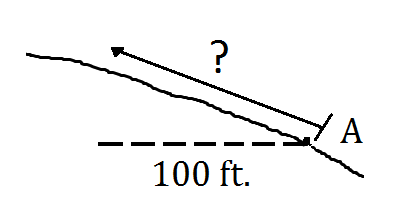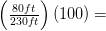# 1.4: Summary Problems

## 1.4 Summary Problems

1. Using the measurements provided, determine the %slope of the following slopes between Points A and B.2. On a 60%slope, Todd wants to walk up a slope a distance equivalent to 100 feet horizontal distance. How far should he walk from Point A?3. On the contour maps below, determine the average slopes between Points A and B. The scale is 1”=2000’. The contour interval is 80’.1.%slope1a.111%

1b.35%

2. On a 60% slope, we know that the rise is 60% of the run. Therefore, the rise here should be 60% of 100 feet or 60 feet. Using the Pythagorean Theorem, we can solve for the hypotenuse.

a2 + b2 = c2 where:

1002 + 602 = c2

13,600 = c2ft.3. The answers to these questions will depend upon how you measured the horizontal, or map distance – hard to do on a screen. My measurements are shown on the maps below:At left. Point A is ≈ 3440’. Point B is ≈ 3720’. The rise is 280’. The run is ≈ 2200’. Therefore, the average slope is (280)(100)/2200 = 13%.

At right. Point A is ≈ 4040’. Point B is ≈ 3280’. The rise is 760’. The run is 1300’. Therefore, the average slope is (760)(100)/2200 = 58%